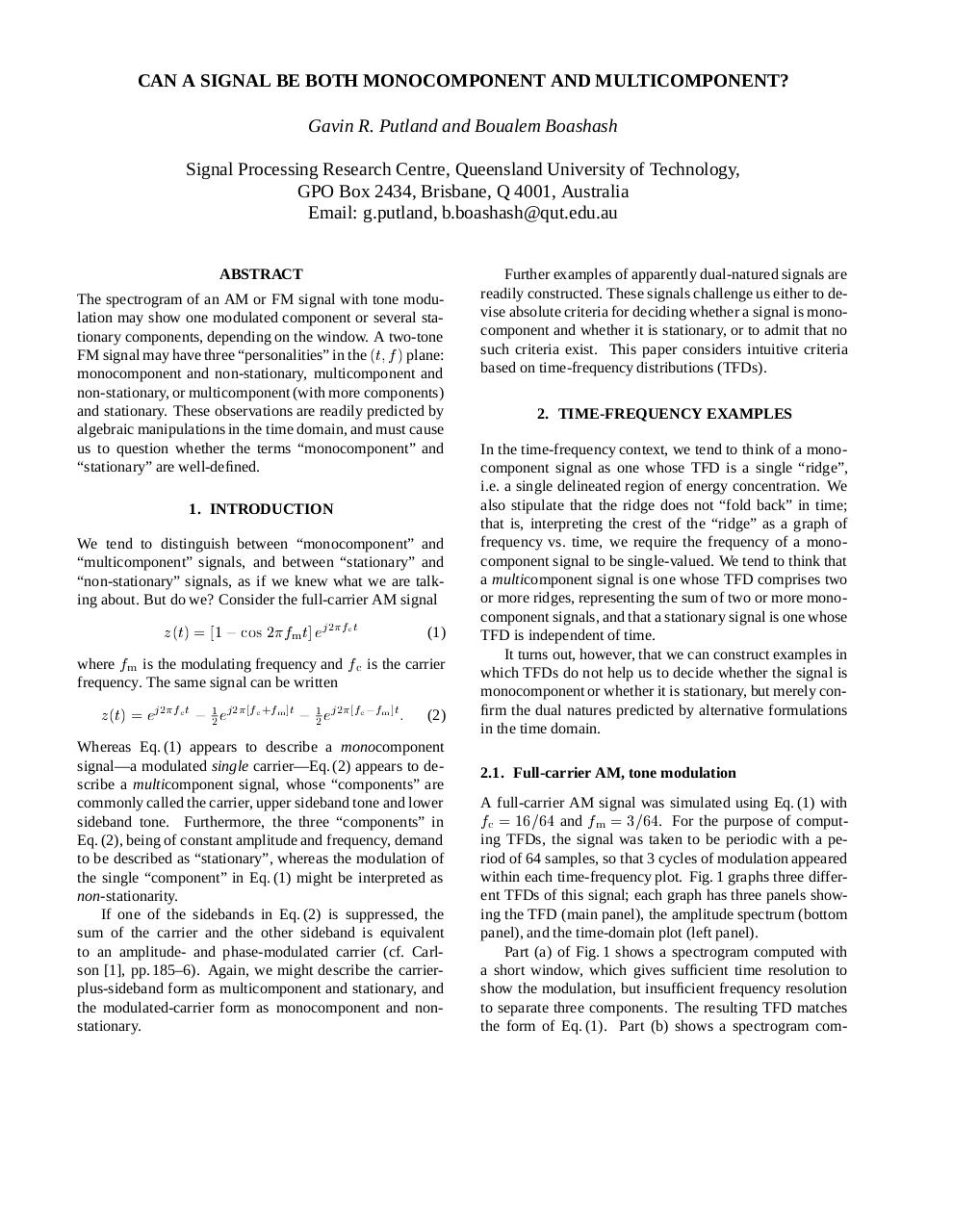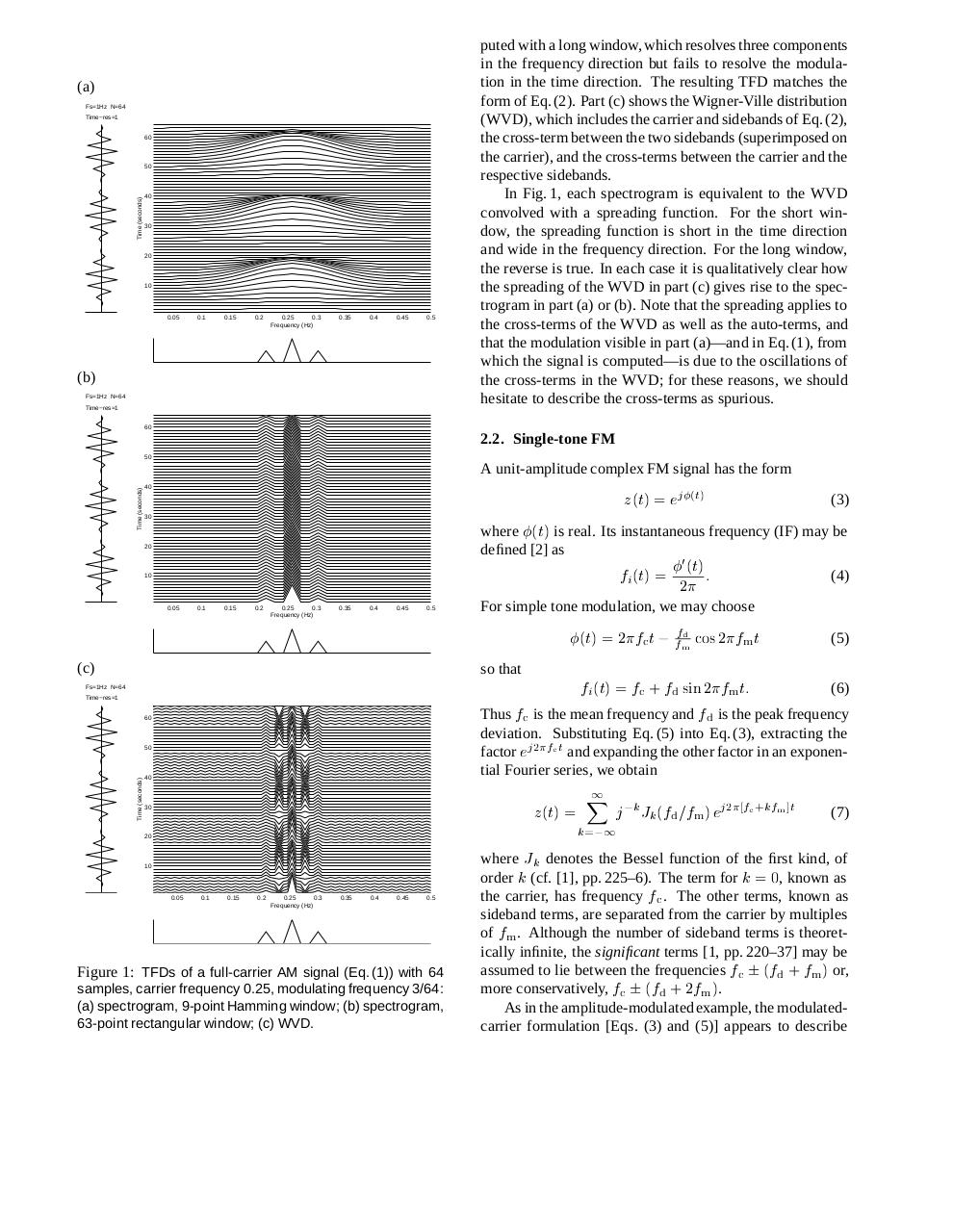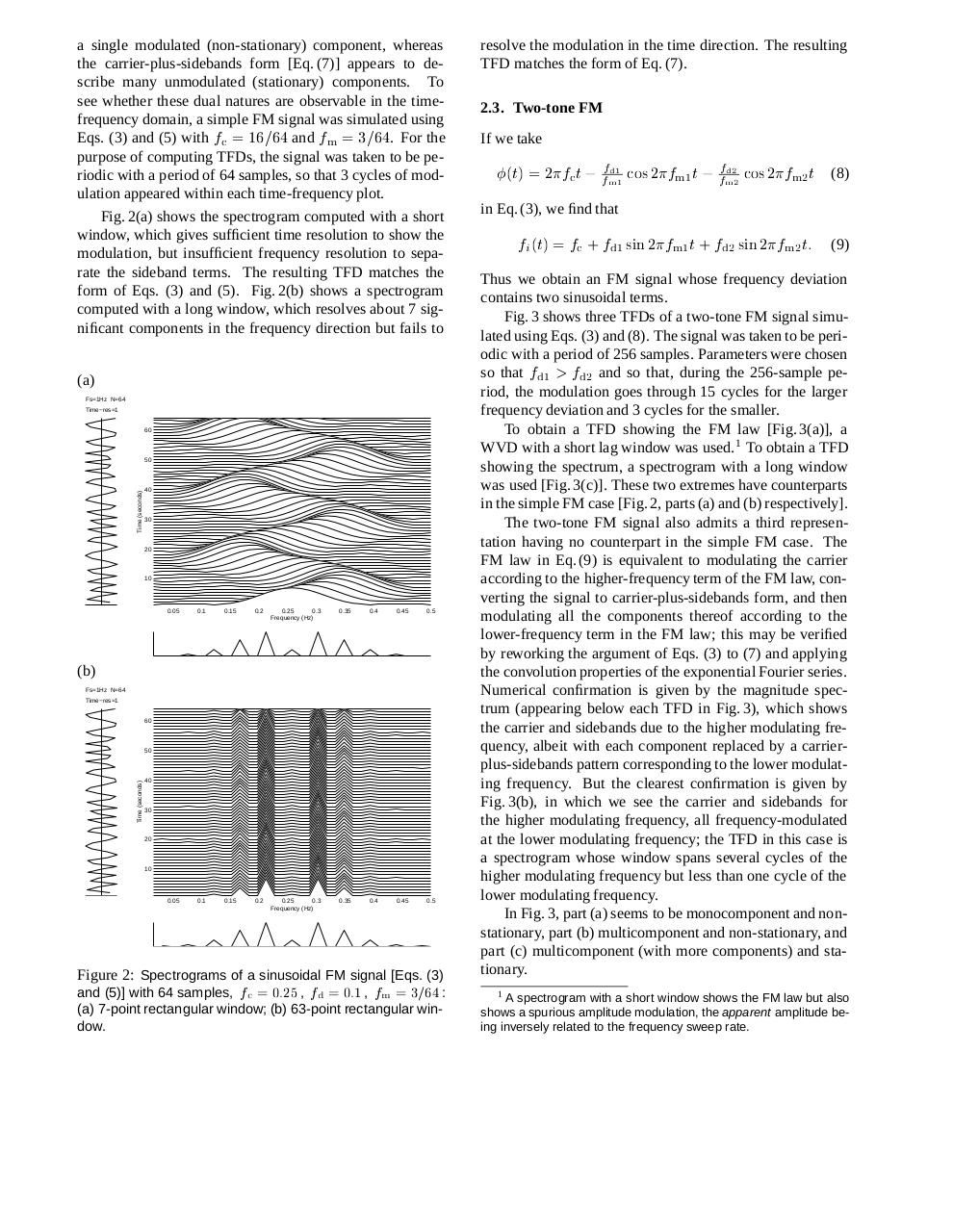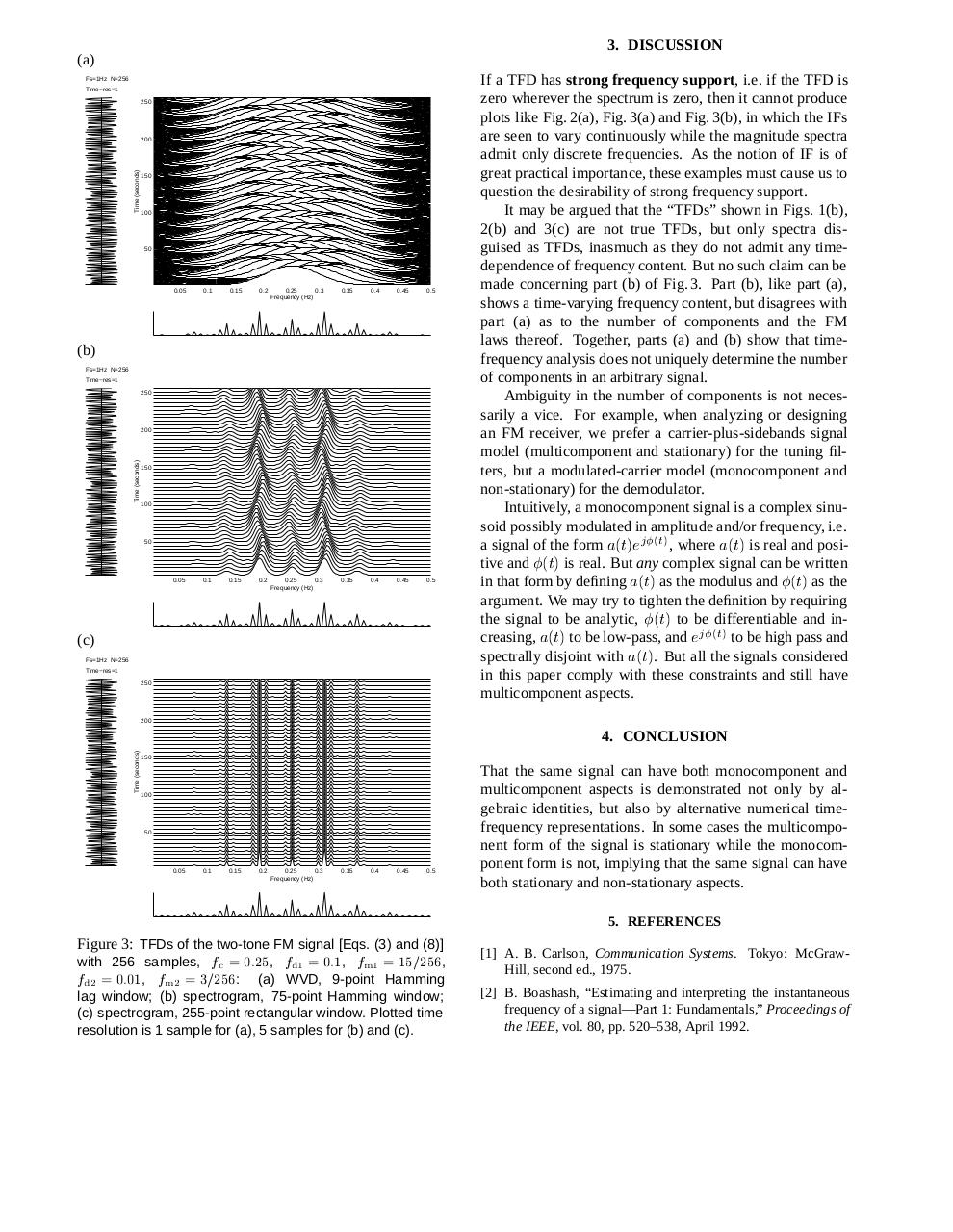# 32 .pdf

### File information

Original filename: 32.pdf

This PDF 1.2 document has been generated by / Acrobat Distiller 4.05 for Windows, and has been sent on pdf-archive.com on 29/05/2016 at 12:21, from IP address 124.149.x.x. The current document download page has been viewed 631 times.
File size: 571 KB (4 pages).
Privacy: public file

32.pdf (PDF, 571 KB)

### Document preview

CAN A SIGNAL BE BOTH MONOCOMPONENT AND MULTICOMPONENT?
Gavin R. Putland and Boualem Boashash
Signal Processing Research Centre, Queensland University of Technology,
GPO Box 2434, Brisbane, Q 4001, Australia
Email: g.putland, b.boashash@qut.edu.au
ABSTRACT
The spectrogram of an AM or FM signal with tone modulation may show one modulated component or several stationary components, depending on the window. A two-tone
FM signal may have three “personalities” in the (t; f ) plane:
monocomponent and non-stationary, multicomponent and
non-stationary, or multicomponent (with more components)
and stationary. These observations are readily predicted by
algebraic manipulations in the time domain, and must cause
us to question whether the terms “monocomponent” and
“stationary” are well-defined.
1. INTRODUCTION
We tend to distinguish between “monocomponent” and
“multicomponent” signals, and between “stationary” and
“non-stationary” signals, as if we knew what we are talking about. But do we? Consider the full-carrier AM signal

z (t) = [1

j 2fc t

cos 2fm t] e

(1)

where fm is the modulating frequency and f c is the carrier
frequency. The same signal can be written

z (t) = ej 2fc t

1
2

ej 2[fc +fm ]t

1
2

ej 2[fc fm ]t :

(2)

Whereas Eq. (1) appears to describe a monocomponent
signal—a modulated single carrier—Eq. (2) appears to describe a multicomponent signal, whose “components” are
commonly called the carrier, upper sideband tone and lower
sideband tone. Furthermore, the three “components” in
Eq. (2), being of constant amplitude and frequency, demand
to be described as “stationary”, whereas the modulation of
the single “component” in Eq. (1) might be interpreted as
non-stationarity.
If one of the sidebands in Eq. (2) is suppressed, the
sum of the carrier and the other sideband is equivalent
to an amplitude- and phase-modulated carrier (cf. Carlson , pp. 185–6). Again, we might describe the carrierplus-sideband form as multicomponent and stationary, and
the modulated-carrier form as monocomponent and nonstationary.

Further examples of apparently dual-natured signals are
readily constructed. These signals challenge us either to devise absolute criteria for deciding whether a signal is monocomponent and whether it is stationary, or to admit that no
such criteria exist. This paper considers intuitive criteria
based on time-frequency distributions (TFDs).
2. TIME-FREQUENCY EXAMPLES
In the time-frequency context, we tend to think of a monocomponent signal as one whose TFD is a single “ridge”,
i.e. a single delineated region of energy concentration. We
also stipulate that the ridge does not “fold back” in time;
that is, interpreting the crest of the “ridge” as a graph of
frequency vs. time, we require the frequency of a monocomponent signal to be single-valued. We tend to think that
a multicomponent signal is one whose TFD comprises two
or more ridges, representing the sum of two or more monocomponent signals, and that a stationary signal is one whose
TFD is independent of time.
It turns out, however, that we can construct examples in
which TFDs do not help us to decide whether the signal is
monocomponent or whether it is stationary, but merely confirm the dual natures predicted by alternative formulations
in the time domain.
2.1. Full-carrier AM, tone modulation
A full-carrier AM signal was simulated using Eq. (1) with

fc = 16=64 and fm = 3=64. For the purpose of computing TFDs, the signal was taken to be periodic with a period of 64 samples, so that 3 cycles of modulation appeared
within each time-frequency plot. Fig. 1 graphs three different TFDs of this signal; each graph has three panels showing the TFD (main panel), the amplitude spectrum (bottom
panel), and the time-domain plot (left panel).
Part (a) of Fig. 1 shows a spectrogram computed with
a short window, which gives sufficient time resolution to
show the modulation, but insufficient frequency resolution
to separate three components. The resulting TFD matches
the form of Eq. (1). Part (b) shows a spectrogram com-

(a)
Fs=1Hz N=64
Time−res=1
60

Time (seconds)

50

40

30

20

10

0.05

0.1

0.15

0.2

0.25
0.3
Frequency (Hz)

0.35

0.4

0.45

0.5

(b)
Fs=1Hz N=64
Time−res=1

puted with a long window, which resolves three components
in the frequency direction but fails to resolve the modulation in the time direction. The resulting TFD matches the
form of Eq. (2). Part (c) shows the Wigner-Ville distribution
(WVD), which includes the carrier and sidebands of Eq. (2),
the cross-term between the two sidebands (superimposed on
the carrier), and the cross-terms between the carrier and the
respective sidebands.
In Fig. 1, each spectrogram is equivalent to the WVD
convolved with a spreading function. For the short window, the spreading function is short in the time direction
and wide in the frequency direction. For the long window,
the reverse is true. In each case it is qualitatively clear how
the spreading of the WVD in part (c) gives rise to the spectrogram in part (a) or (b). Note that the spreading applies to
the cross-terms of the WVD as well as the auto-terms, and
that the modulation visible in part (a)—and in Eq. (1), from
which the signal is computed—is due to the oscillations of
the cross-terms in the WVD; for these reasons, we should
hesitate to describe the cross-terms as spurious.

60

2.2. Single-tone FM
50

Time (seconds)

A unit-amplitude complex FM signal has the form

z (t) = ej(t)

40

30

(3)

where (t) is real. Its instantaneous frequency (IF) may be
defined  as

20

fi (t) =

10

0.05

0.1

0.15

0.2

0.25
0.3
Frequency (Hz)

0.35

0.4

0.45

0.5

(4)

For simple tone modulation, we may choose

(t) = 2fc t
(c)

0 (t)
:
2

fd
fm cos 2fm t

(5)

so that

fi (t) = fc + fd sin 2fm t:
(6)
Thus fc is the mean frequency and f d is the peak frequency

Fs=1Hz N=64
Time−res=1
60

deviation. Substituting Eq. (5) into Eq. (3), extracting the
factor ej 2fc t and expanding the other factor in an exponential Fourier series, we obtain

Time (seconds)

50

40

z (t) =

30

20

10

0.05

0.1

0.15

0.2

0.25
0.3
Frequency (Hz)

0.35

0.4

0.45

0.5

Figure 1: TFDs of a full-carrier AM signal (Eq. (1)) with 64
samples, carrier frequency 0.25, modulating frequency 3/64:
(a) spectrogram, 9-point Hamming window; (b) spectrogram,
63-point rectangular window; (c) WVD.

X1

k=

1

j k Jk (fd =fm ) ej 2[fc +kfm ]t

(7)

where Jk denotes the Bessel function of the first kind, of
order k (cf. , pp. 225–6). The term for k = 0, known as
the carrier, has frequency f c . The other terms, known as
sideband terms, are separated from the carrier by multiples
of fm . Although the number of sideband terms is theoretically infinite, the significant terms [1, pp. 220–37] may be
assumed to lie between the frequencies f c  (fd + fm ) or,
more conservatively, f c  (fd + 2fm ).
As in the amplitude-modulated example, the modulatedcarrier formulation [Eqs. (3) and (5)] appears to describe

a single modulated (non-stationary) component, whereas
the carrier-plus-sidebands form [Eq. (7)] appears to describe many unmodulated (stationary) components. To
see whether these dual natures are observable in the timefrequency domain, a simple FM signal was simulated using
Eqs. (3) and (5) with f c = 16=64 and fm = 3=64. For the
purpose of computing TFDs, the signal was taken to be periodic with a period of 64 samples, so that 3 cycles of modulation appeared within each time-frequency plot.
Fig. 2(a) shows the spectrogram computed with a short
window, which gives sufficient time resolution to show the
modulation, but insufficient frequency resolution to separate the sideband terms. The resulting TFD matches the
form of Eqs. (3) and (5). Fig. 2(b) shows a spectrogram
computed with a long window, which resolves about 7 significant components in the frequency direction but fails to
(a)
Fs=1Hz N=64
Time−res=1
60

Time (seconds)

50

40

30

20

10

0.05

0.1

0.15

0.2

0.25
0.3
Frequency (Hz)

0.35

0.4

0.45

0.5

0.05

0.1

0.15

0.2

0.25
0.3
Frequency (Hz)

0.35

0.4

0.45

0.5

(b)
Fs=1Hz N=64
Time−res=1
60

Time (seconds)

50

40

30

20

10

Figure 2: Spectrograms of a sinusoidal FM signal [Eqs. (3)
and (5)] with 64 samples, f c = 0:25 , fd = 0:1 , fm = 3=64 :
(a) 7-point rectangular window; (b) 63-point rectangular window.

resolve the modulation in the time direction. The resulting
TFD matches the form of Eq. (7).
2.3. Two-tone FM
If we take
fd1
fm1 cos 2fm1 t

fd2
fm2 cos 2fm2 t

(8)

fi (t) = fc + fd1 sin 2fm1 t + fd2 sin 2fm2 t:

(9)

(t) = 2fc t

in Eq. (3), we find that

Thus we obtain an FM signal whose frequency deviation
contains two sinusoidal terms.
Fig. 3 shows three TFDs of a two-tone FM signal simulated using Eqs. (3) and (8). The signal was taken to be periodic with a period of 256 samples. Parameters were chosen
so that fd1 &gt; fd2 and so that, during the 256-sample period, the modulation goes through 15 cycles for the larger
frequency deviation and 3 cycles for the smaller.
To obtain a TFD showing the FM law [Fig. 3(a)], a
WVD with a short lag window was used. 1 To obtain a TFD
showing the spectrum, a spectrogram with a long window
was used [Fig. 3(c)]. These two extremes have counterparts
in the simple FM case [Fig. 2, parts (a) and (b) respectively].
The two-tone FM signal also admits a third representation having no counterpart in the simple FM case. The
FM law in Eq. (9) is equivalent to modulating the carrier
according to the higher-frequency term of the FM law, converting the signal to carrier-plus-sidebands form, and then
modulating all the components thereof according to the
lower-frequency term in the FM law; this may be verified
by reworking the argument of Eqs. (3) to (7) and applying
the convolution properties of the exponential Fourier series.
Numerical confirmation is given by the magnitude spectrum (appearing below each TFD in Fig. 3), which shows
the carrier and sidebands due to the higher modulating frequency, albeit with each component replaced by a carrierplus-sidebands pattern corresponding to the lower modulating frequency. But the clearest confirmation is given by
Fig. 3(b), in which we see the carrier and sidebands for
the higher modulating frequency, all frequency-modulated
at the lower modulating frequency; the TFD in this case is
a spectrogram whose window spans several cycles of the
higher modulating frequency but less than one cycle of the
lower modulating frequency.
In Fig. 3, part (a) seems to be monocomponent and nonstationary, part (b) multicomponent and non-stationary, and
part (c) multicomponent (with more components) and stationary.
1 A spectrogram with a short window shows the FM law but also
shows a spurious amplitude modulation, the apparent amplitude being inversely related to the frequency sweep rate.

3. DISCUSSION
(a)
Fs=1Hz N=256
Time−res=1
250

Time (seconds)

200

150

100

50

0.05

0.1

0.15

0.2

0.25
0.3
Frequency (Hz)

0.35

0.4

0.45

0.5

0.05

0.1

0.15

0.2

0.25
0.3
Frequency (Hz)

0.35

0.4

0.45

0.5

(b)
Fs=1Hz N=256
Time−res=1
250

Time (seconds)

200

150

100

50

(c)
Fs=1Hz N=256
Time−res=1
250

If a TFD has strong frequency support, i.e. if the TFD is
zero wherever the spectrum is zero, then it cannot produce
plots like Fig. 2(a), Fig. 3(a) and Fig. 3(b), in which the IFs
are seen to vary continuously while the magnitude spectra
admit only discrete frequencies. As the notion of IF is of
great practical importance, these examples must cause us to
question the desirability of strong frequency support.
It may be argued that the “TFDs” shown in Figs. 1(b),
2(b) and 3(c) are not true TFDs, but only spectra disguised as TFDs, inasmuch as they do not admit any timedependence of frequency content. But no such claim can be
made concerning part (b) of Fig. 3. Part (b), like part (a),
shows a time-varying frequency content, but disagrees with
part (a) as to the number of components and the FM
laws thereof. Together, parts (a) and (b) show that timefrequency analysis does not uniquely determine the number
of components in an arbitrary signal.
Ambiguity in the number of components is not necessarily a vice. For example, when analyzing or designing
an FM receiver, we prefer a carrier-plus-sidebands signal
model (multicomponent and stationary) for the tuning filters, but a modulated-carrier model (monocomponent and
non-stationary) for the demodulator.
Intuitively, a monocomponent signal is a complex sinusoid possibly modulated in amplitude and/or frequency, i.e.
a signal of the form a(t)e j(t) , where a(t) is real and positive and (t) is real. But any complex signal can be written
in that form by defining a(t) as the modulus and (t) as the
argument. We may try to tighten the definition by requiring
the signal to be analytic, (t) to be differentiable and increasing, a(t) to be low-pass, and e j(t) to be high pass and
spectrally disjoint with a(t). But all the signals considered
in this paper comply with these constraints and still have
multicomponent aspects.

200

Time (seconds)

4. CONCLUSION
150

100

50

0.05

0.1

0.15

0.2

0.25
0.3
Frequency (Hz)

0.35

0.4

0.45

0.5

That the same signal can have both monocomponent and
multicomponent aspects is demonstrated not only by algebraic identities, but also by alternative numerical timefrequency representations. In some cases the multicomponent form of the signal is stationary while the monocomponent form is not, implying that the same signal can have
both stationary and non-stationary aspects.
5. REFERENCES

Figure 3: TFDs of the two-tone FM signal [Eqs. (3) and (8)]
with 256 samples, f c = 0:25, fd1 = 0:1, fm1 = 15=256 ,
fd2 = 0:01,
fm2 = 3=256 :
(a) WVD, 9-point Hamming
lag window; (b) spectrogram, 75-point Hamming window;
(c) spectrogram, 255-point rectangular window. Plotted time
resolution is 1 sample for (a), 5 samples for (b) and (c).

 A. B. Carlson, Communication Systems. Tokyo: McGrawHill, second ed., 1975.
 B. Boashash, “Estimating and interpreting the instantaneous
frequency of a signal—Part 1: Fundamentals,” Proceedings of
the IEEE, vol. 80, pp. 520–538, April 1992.#### HTML Code

Copy the following HTML code to share your document on a Website or Blog

#### QR Code### Related keywords